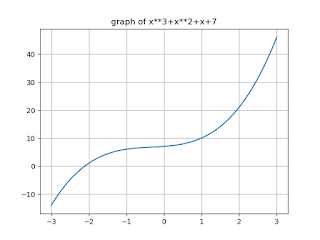# Regula Falsi Method in Python

In this, you will learn Regula Falsi Methon implementation in Python Programming. First of all, we discuss Regula falsi method and try to understand the concept of Regula Falsi. In the next part you will learn how to write code in Python.

### Regula falsi method or method of false position

Regula falsi method or method of false position is a numerical method to solve an unknown equation. This is very similar to the bisection method algorithm and is one of the oldest methods. The bisection method was developed so that it converges at a very slow speed.

The process of convergence in the bisection method is very slow. It depends on the choice of end points of the interval [a, b]. The f (x) function has no role in finding point C (which is the point between a and b).

It can only be used to determine the next short interval [a, c] or [c, b]. C can be better estimated by taking a straight line L connecting the points (a, f (a)) and (b, f (b)) that meet the x-axis. To obtain the value of c we can equate the two expressions of the slope m of the L line.

This method is an improvement over the slow convergence of the bisection method. To find the source, the input consists of a search interval [a, b], followed by a tangent joining (a, f (a)) & (b, f (b)). The point at which the tangent touches the x-axis is an interesting point.

#### Steps:

The continuous function f (x) is given at intervals [a, b] such as F (a) * f (b) <0

c = (a * f (b) - b * f (a)) / (f (b) - f (a))

f (a) * f (c) <0

if b = c

else a = c

#### Example 1: Write a Program to find and print roots of the given equation x**3-x**2+2 by Regula Falsi Method

Python code:

`max_iter=100000def func(x):    return x**3-x**2+2a = -100b = 200def regulafalsi(a,b):    if func(a)*func(b)>=0:        print("a and b are not rightly assumed")    return 1c = afor i in range(max_iter):    c = (a*func(b)-(b*func(a)))/(func(b)-func(a))    if func(c)==0:        break    elif func(a)*func(c)<0:        b = c    else:        a = cprint("root of equation is:",c)regulafalsi(a,b)`
`Output:`
```root of equation is: -1.0000028705371073
```

#### Example 2: To print the root of equation x**3+x**2+x+7 and plot its graph

Python Code:
`from pylab import *import mathdef f(x):    y = x**3+x**2+x+7    return yx = linspace(-3,3,100)xa = -1xb = -3xc = (xa*f(xb)-(xb*f(xa)))/(f(xb)-f(xa))while abs(f(xc))>0.001:    if f(xc)*f(xa)>0:        xa = xc    else:        xb = xc    xc = (xa*f(xb)-(xb*f(xa)))/(f(xb)-f(xa))print("The root is:",xc)plot(x,f(x))title("graph of x**3+x**2+x+7")grid(True)show()`
`Output:`
```The root is: -2.104817327832827
```#### Example 3: To print roots of x3-2x+1 and plot its graph

Python Code:
`from pylab import *import mathdef f(x):    y = x**3+2*x+1    return yx = linspace(-3,3,100)xa = -1xb = -2xc = (xa*f(xb)-(xb*f(xa)))/(f(xb)-f(xa))while abs(f(xc))>0.01:    if f(xc)*f(xa)>0:        xa = xc    else:        xb = xc    xc = (xa*f(xb)-(xb*f(xa)))/(f(xb)-f(xa))print("The root is:",xc)plot(x,f(x))title("graph of x**3+2*x+1")grid(True)show()`
`output:`
```The root is: -0.4564692206092183
```